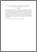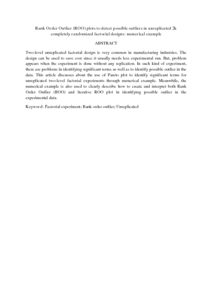# Rank order outlier (ROO) plots to detect possible outliers in unreplicated 2k completely randomized factorial designs: numerical example

## Citation

Fitrianto, Anwar and Haur, Low Chuan (2016) Rank order outlier (ROO) plots to detect possible outliers in unreplicated 2k completely randomized factorial designs: numerical example. ARPN Journal of Engineering and Applied Sciences, 11 (18). pp. 10775-10779. ISSN 1819-6608

## Abstract

Two-level unreplicated factorial design is very common in manufacturing industries. The design can be used to save cost since it usually needs less experimental run. But, problem appears when the experiment is done without any replication. In such kind of experiment, there are problems in identifying significant terms as well as to identify possible outlier in the data. This article discusses about the use of Pareto plot to identify significant terms for unreplicated two-level factorial experiments through numerical example. Meanwhile, the numerical example is also used to clearly describe how to create and interpret both Rank Order Outlier (ROO) and iterative ROO plot in identifying possible outlier in the experimental data.Preview
PDF
Rank Order Outlier (ROO) plots to detect possible outliers in unreplicated 2k completely randomized factorial designs.pdfView Item